# How To Work Out Flop Probability In Texas Holdem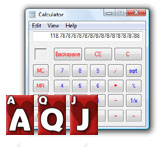In the previous article on working out preflop hand probability, we worked out the likelihood of being dealt different combinations of starting hands before the flop.

In this article, I will cover the basics of working out the probabilities behind various possible flops. I'll go ahead and cover the probability basics first in case you missed it in the preflop probability article.

## A few probability basics.

When working out flop probabilities, the main probabilities we will work with are the number of cards left in the deck and the number of cards we want to be dealt on the flop. So for example, if we were going to deal out 1 card:

• The probability of dealing a 7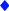would be 1/52 - There is one 7in a deck of 52 cards.
• The probability of dealing any Ace would be 4/52 - There four Aces in a deck of 52 cards.
• The probability of dealing any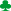would be 13/52 - There are 13s in a deck of 52 cards.

In fact, the probability of being dealt any random card (not just the 7) would be 1/52. This also applies to the probability being dealt any random value of card like Kings, tens, fours, whatever (4/52) and the probability of being dealt any random suit (13/52).

Each card is just as likely to be dealt as any other - no special priorities in this game!

### The numbers change for future cards.

A quick example... let's say we want to work out the probability of being dealt a pair of sevens.

• The probability of being dealt a 7 for the first card will be 4/52.
• The probability of being dealt a 7 for the second card will be 3/51.

Notice how the probability changes for the second card? After we have been dealt the first card, there is now 1 less card in the deck making it 51 cards in total. Also, after already being dealt a 7, there are now only three 7s left in the deck.

Always try and take care with the numbers for future cards. The numbers will change slightly as you go along.

### Working out probabilities.

• Whenever the word "and" is used, it will usually mean multiply.
• Whenever the word "or" is used, it will usually mean add.

This won't make much sense for now, but it will make a lot of sense a little further on in the article. Trust me.

## Total number of flop combinations.

First of all, lets work out the total number of possible flop combinations. In other words, we will just be working out the probability of "any random flop".

To work out this probability we simply multiply the probability of 3 individual cards being dealt.

• (random card) * (random card) * (random card)
• (1/52) * (1/51) * (1/50) = 132,600 possible flops.

Pretty big combination of cards huh? However, we've omitted the fact that we know our 2 holecards, so there will be two less known cards in the deck when we are dealing the flop. So if we amend this calculation by starting at 50 instead of 52:

P = (1/50) * (1/49) * (1/48)
P = 1/117,600.

Better, but this 1/117,600 probability is with exact cards in order. In Texas Hold'em it does not make a difference whether the flop comes AKQ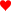or AQK. So to account for this we multiply this fraction by 6 (1*2*3 = 6).

P = 1/117,600 * 6
P = 1/19,600.

The order of cards on the flop makes no difference, so multiply the probability by 6 to account for this (1 * 2 * 3 = 6 - this is math probability stuff). Don't worry if you don't know why we do this, just take it as it is.

This means that the probability of the flop being AKQin any order is 1/19,600 - which is exactly the same probability as the flop coming something like 2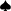59in any order.

So in total there are 19,600 different possible flops in Texas Hold'em.

## Probability of specific flops.

To work out the probability of specific flops with the cards in any order we simply multiply the probabilities of each of those cards being dealt.

Multiply the 3 probabilities together.

So let's say we want to find the probability of flopping a heart flush.

• There are 2 hearts in our hand.
• There are 11 hearts left in a deck of 50.

P = (11/50) * (10/49) * (9/48)
P = 990/117600 = 1/119

In this example we do not need to multiply the final probability by 6. This is because the order of the cards and their probabilities are important, as the overall probabilities decrease as each heart is dealt.

## Overview of working out flop probabilities.

This is a really basic article for working out flop probabilities in Texas Hold'em. Think of it as more of a taster for working out probabilities on the flop to help you get your feet wet.

If I were to continue with probabilities, I would be delving deeper in to mathematics and further away from poker. That wouldn't necessarily be a bad thing, but my maths is a bit rusty and it's not going to directly improve your game, so I'll stop for now. Maybe I'll address it in a future article, but for now this is as far as I'm going to go.

If you're really interested in the mathematics of the game, try the book: The Mathematics of Poker by Bill Chen. It's definitely not a light read, but it's very interesting if you enjoy the maths side of the game. For other books, try the poker books section.

### Other useful articles.

Go back to the poker odds charts.

How Much More Money Could
You Be Winning?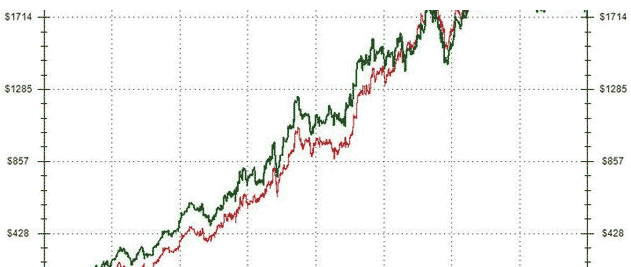“I played break-even online poker for 4 years before finding
DeucesCracked, for the last 5 months I've made more money playing
poker than at my full-time job.”
- liquid_quik, DC Member

# Subscribe to thepokerbank

I'll send you an email if I add something new and interesting to the website.

Don't worry, it doesn't happen very often.